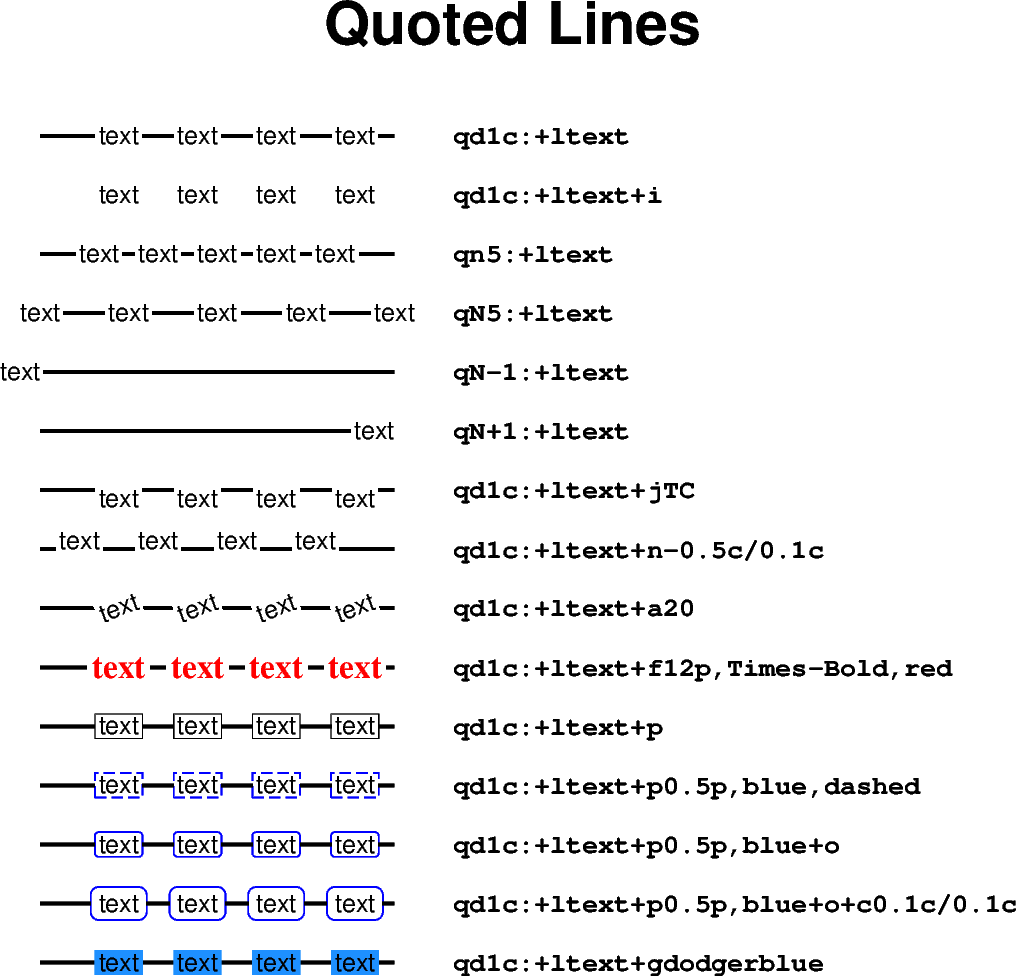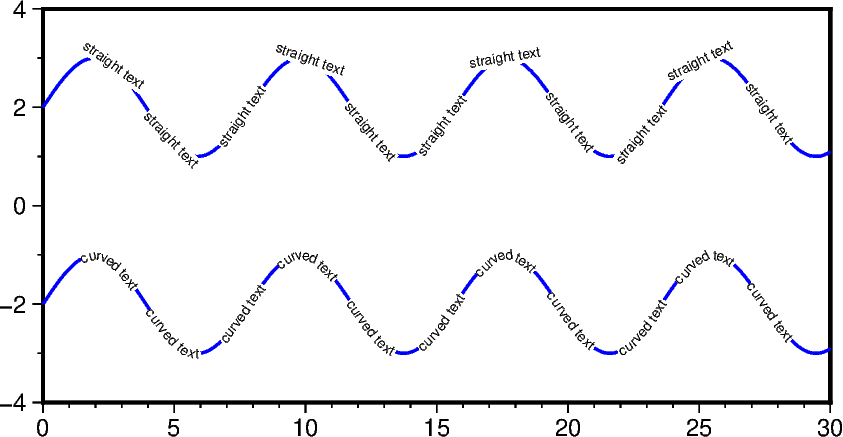# Quoted lines

To plot a so-called quoted line, i.e., labels along a line or curve, use the `style` parameter of the `pygmt.Figure.plot` method with the argument `"q"` and the desired modifiers. A colon (`":"`) is used to separate the algorithm settings from the label information. This example shows how to adjust the labels. For modifying the main quoted line via the `pen` parameter, see the Line styles example. For details on the input data see the upstream GMT documentation at https://docs.generic-mapping-tools.org/latest/plot.html#s.

```import numpy as np
import pygmt

# Generate a two-point line for plotting
x = np.array([1, 4])
y = np.array([20, 20])

fig = pygmt.Figure()
fig.basemap(region=[0, 10, 0, 20], projection="X15c/15c", frame="+tQuoted Lines")

# Plot different quoted lines
for quotedline in [
# Line with labels ("+l") "text" in distance ("d") of 1 centimeter
"qd1c:+ltext",
# Suppress the main quoted line by appending "+i"
"qd1c:+ltext+i",
# Give the number of equally spaced labels by using "n" instead of "d"
"qn5:+ltext",
# Use upper-case "N" to have labels at the start and end of the line
"qN5:+ltext",
# To only plot a label at the start of the line use "N-1"
"qN-1:+ltext",
# To only plot a label at the end of the line use "N+1"
"qN+1:+ltext",
# Adjust the justification of the labels via "+j", here Top Center
"qd1c:+ltext+jTC",
# Shift labels using "+n" in x and y directions relative to the main
# quoted line
"qd1c:+ltext+n-0.5c/0.1c",
# Rotate labels via "+a" (counter-clockwise from horizontal)
"qd1c:+ltext+a20",
# Adjust size, type, and color of the font via "+f"
"qd1c:+ltext+f12p,Times-Bold,red",
# Add a box around the label via "+p"
"qd1c:+ltext+p",
# Adjust thickness, color, and style of the outline
"qd1c:+ltext+p0.5p,blue,dashed",
# Append "+o" to get a box with rounded edges
"qd1c:+ltext+p0.5p,blue+o",
# Adjust the space between label and box in x and y directions via "+c"
"qd1c:+ltext+p0.5p,blue+o+c0.1c/0.1c",
# Give a fill of the box via "+g" together with the desired color
"qd1c:+ltext+gdodgerblue",
]:
y -= 1  # Move current line down
fig.plot(x=x, y=y, pen="1.25p", style=quotedline)
fig.text(
x=x[-1],
y=y[-1],
text=quotedline,
font="Courier-Bold",
justify="ML",
offset="0.75c/0c",
)

fig.show()
```For curved labels following the line, append `"+v"` to the argument passed to the `style` parameter.

```# Generate sinus curve
x = np.arange(0, 10 * np.pi, 0.1)
y = np.sin(0.8 * x)

fig = pygmt.Figure()

fig.basemap(region=[0, 30, -4, 4], projection="X10c/5c", frame=True)

fig.plot(x=x, y=y + 2, style="qd1.2c:+lstraight text+f5p", pen="1p,blue")

fig.plot(
x=x,
y=y - 2,
# Append "+v" to force curved labels
style="qd1.2c:+lcurved text+f5p+v",
pen="1p,blue",
)

fig.show()
```Total running time of the script: ( 0 minutes 4.888 seconds)

Gallery generated by Sphinx-Gallery